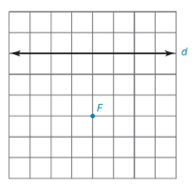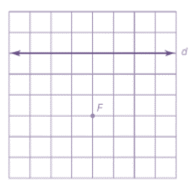Chapter 7.1, Problem 24EElementary Geometry For College St...

7th Edition
Alexander + 2 others
ISBN: 9781337614085

Solutions

Chapter
SectionElementary Geometry For College St...

7th Edition
Alexander + 2 others
ISBN: 9781337614085
Textbook Problem

Note: Exercises preceded by an asterisk are of a more challenging nature.For Exercises 23 to 28, use the grid and your compass (as needed) to locate several points on the parabola having the given focus F and directrix d . Then sketch the parabola that is characterized by these points.To determine

To sketch:

The parabola using the below given grid with directrix and focus.Explanation

Definition:

A parabola is the locus of points that are equidistant from a fixed line and a fixed point not on that line.

The fixed point is known as focus of the parabola and the fixed line is known as directrix of the parabola.

Procedure:

1. Draw the given directrix d and focus F and locate a point D1.

2. Locate a point P1 which is the mid-point of FD1. The point P1 will be the vertex.

3. Locate the points P1, P2, P3, P4, and P5 such that D1P1=P1F, D2<

Still sussing out bartleby?

Check out a sample textbook solution.

See a sample solution

The Solution to Your Study Problems

Bartleby provides explanations to thousands of textbook problems written by our experts, many with advanced degrees!

Get Started

If f(x)=0sinx1+t2dt and g(y)=3yf(x)dx, find g(/6).

Single Variable Calculus: Early Transcendentals, Volume I

What is the slope of a nonvertical line? What can you say about the slope of a vertical line?

Applied Calculus for the Managerial, Life, and Social Sciences: A Brief Approach

Evaluate the expression sin Exercises 116. (23)2

Finite Mathematics and Applied Calculus (MindTap Course List)

The point P graphed at the right has spherical coordinates:

Study Guide for Stewart's Multivariable Calculus, 8th